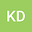A mathematical study of basic reproduction R0 and its significance in controlling of corona-virus (COVID-19) disease transmission
•• Krishna Das
Krishna DasThe basic reproduction number of an infectious agent is the average number of infections one case can generate over the course of the infectious period, in a naive, uninfected population. This primer article focuses on the basic reproduction number, R0, for infectious diseases, and other reproduction numbers related to $R_0$ that are useful in guiding control strategies. Beginning with a simple population model, the concept is developed for a threshold value of R0 determining whether or not the disease dies out. The next generation matrix method of calculating R0 in a compartmental model is described and illustrated. To address control strategies reproduction numbers are defined and these theoretical ideas are then applied to models that are formulated for different SI, SI with incubation delay, SIR, SEIR and SEQIR the novel coronavirus (2019-nCoV) infection model , the reproduction number has been found to vary, reflecting the dynamics of transmission of the coronavirus outbreak as well as the case reporting rate. If $R_0 > 1,$ then the number of latently infected individuals exponentially grows. However, if $R_0 < 1$ (e.g. due to quarantine measures and contact restrictions imposed by public authorities), then the number of infected decays exponentially. We then consider the available data on the disease development in different countries to show that there are three possible patterns: growth dynamics, growth- decays dynamics, and patchy dynamics. During this period of time, the growth rate of the total number of infected was gradually decreasing.